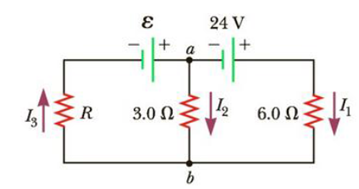Chapter 18, Problem 22P

Chapter
Section
Textbook Problem

In the circuit of Figure P18.22, the current I1 is 3.0 A and the values of ε and R are unknown. What are the currents I2 and I3?Figure P18.22

To determine
The current I2 and I3 in the circuit.

Explanation

Given Info: Voltage ε1 is 24V and Resistance R1 is 6.0Ω , Current I1 is 3.0A , Resistance R2 is 3.0Ω , Change in potential VbVa is 6.0V .

Explanation:

Formula to calculate the change in potential

Follow the path of I1 from a to b

VbVa=ε1R1I1

• ε1 is voltage in the circuits
• R1 is resistance in the circuits
• I1 is current in the circuits
• Va voltage at point a
• Vb voltage at point b

Substitute 24V for ε1 , 6.0Ω for R1 and 3.0A for I1 in the above equation to find VbVa

VbVa=24V(6.0Ω)(3.0Ω)=6.0V

Formula to calculate the current I2

Follow the path of I2 from a to b

VbVa=R2I2

• R2 is corresponding resistance in the circuits
• I2 is current in the circuits
• Va voltage at point a
• Vb voltage at point b

Substitute 3

Still sussing out bartleby?

Check out a sample textbook solution.

See a sample solution

The Solution to Your Study Problems

Bartleby provides explanations to thousands of textbook problems written by our experts, many with advanced degrees!

Get Started

Find more solutions based on key concepts